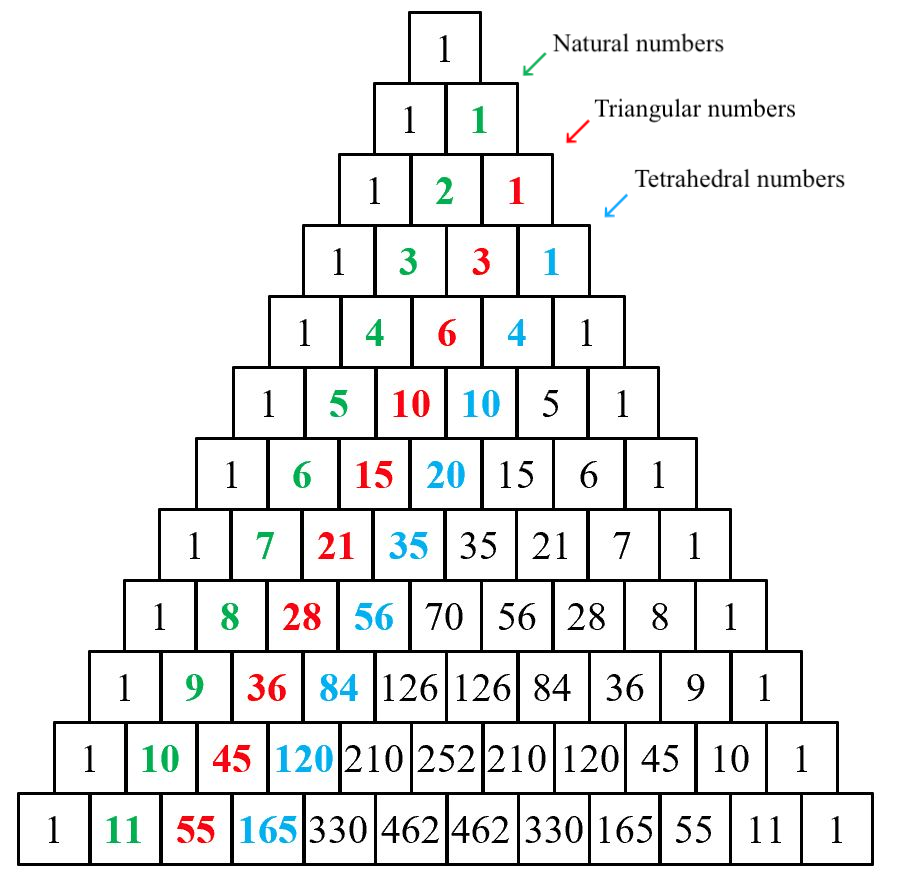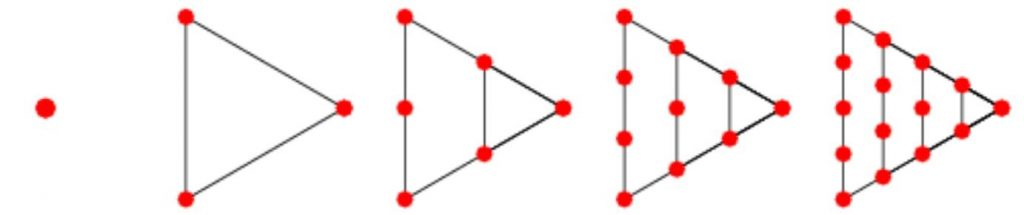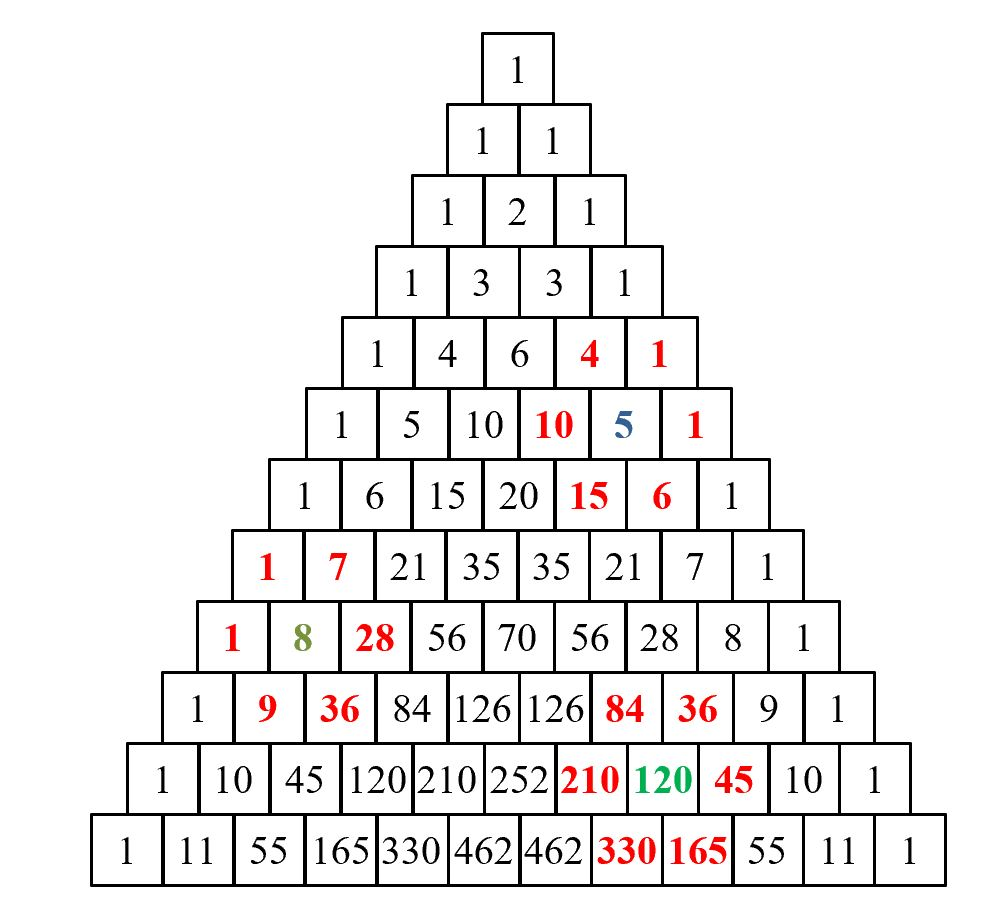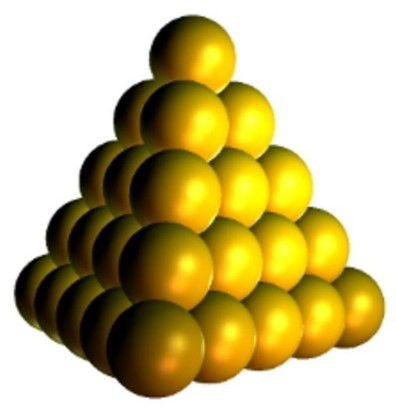# Sequences in the triangle and the fourth dimension

This is the second in a series of guest posts by David Benjamin, exploring the secrets of Pascal’s Triangle.

## Sequences in the diagonals

There are many sequences of numbers to be found in Pascal’s triangle. The Natural numbers occur in the second diagonal, running in either direction, and the next two diagonals after that contain other important sequences:The set of Natural numbers are in the second diagonal, the set of triangular numbers in the third diagonal and the set of tetrahedral numbers in the fourth diagonal

The triangular numbers are part of the sequence of polygonal numbers, all of which can be displayed geometrically by a series of dots. All sets of polygonal numbers begin with $1$ – representing the degenerate polygon, which isn’t really a polygon.$1, 3, 6, 10, 15, 21,…$

The third diagonal in Pascal’s triangle contains the set of triangular numbers.

Two congruent right-angled triangles can be joined to create a square. In a similar way, summing successive pairs of triangular numbers creates the set of square numbers and so the sequence of square numbers can also be considered to be contained in the third diagonal.

{$1 + 3, 3 + 6, 6 +10, 10 +15, 15 + 21,…$} = {$1, 4, 9, 16, 25, 36,…$}

Yang Hui discovered the formula to find the sum of the triangular numbers. The $nth$ term for the sequence is $\frac{n(n+1)}{2}$ and the sum of the first $n$ terms is $\frac{n(n+1)(n+2)}{6}$, where each term or sum is found by substituting $n = 1, 2, 3, 4,..$

## More Square numbers

Square numbers can also be found in the triangle by calculating the product of the six numbers surrounding any internal number:The product of the six numbers surrounding any internal number produces a square number

$4\times 1\times 1\times 6\times 15\times 10=3 \hspace{0.1cm} 600=60^2$

$84\times 210\times 330\times 165\times 45\times 36=1 \hspace{0.1cm} 556 \hspace{0.1cm} 006 \hspace{0.1cm} 760 \hspace{0.1cm} 000=1 \hspace{0.1cm} 247 \hspace{0.1cm} 400^2$

$1\times 7\times 28\times 36\times 9\times 1=63 \hspace{0.1cm} 504=252^2$

## Higher dimensional numbers

The fourth diagonal of the triangle contains the sequence of tetrahedral numbers, part of the set of pyramidal numbers. They can be represented in 3 dimensions and are created from the triangular numbers.

$1$ on top with $3$ underneath, then $6$, then $10$,….They are displayed below as a stack of balls representing a triangular-based prism. They can also be generated by the power series $\displaystyle\frac{x}{(x-1)^4}=x+4x^2+10x^3+20x^4+35x^5+56x^6+…$. Conway and Guy in their wonderful book, The Book of Numbers, wrote that the tetrahedral numbers are all even except every fourth one, which is odd.The first 5 tetrahedral numbers represented as a triangular prism

The fifth diagonal of the triangle contains the pentatope numbers: $1, 5, 15, 35, 70, 126, 210,..$ Pascal called them the triangulo-triangular numbers. Pentatope numbers are the sums of the tetrahedral numbers which are the sums of the triangular numbers. The $nth$ term for the sequence is $\frac{n(n+1)(n+2)(n+3)}{24}$ which tells us that the product of four consecutive numbers is divisible by $24$. The power series to generate them is $\frac{x}{(x-1)^5}=x+5x^2+15x^3+35x^4+70x^5+126x^6+…$.

Wolfram Mathworld describes the pentatope as the simplest regular figure in four dimensions, representing the four-dimensional analog of the solid tetrahedron. It is also called the 5-cell, since it consists of five vertices, or pentachoron. The pentatope is the four-dimensional simplex.

The power series $\frac{x}{(x-1)^n}$ generate the diagonals of Pascal’s triangle for $n=1,2,3,4,5,…$

## Some other patterns

In Part 1 we saw that the numbers in each row sum to the powers of $2$. If we concatenate the numbers in the first five rows of the triangle then we obtain the powers of $11$:

Now with some reverse concatenation and summing some pairs of digits we obtain further powers…

$1 5 10 10 5 1 \rightarrow 1 (5 + 1) (0 + 1) 0 5 1 \rightarrow 161051 = 11^5$

$1 6 15 20 15 6 1 \rightarrow 1 (6 + 1) (5 + 2) (0 + 1) 5 6 1 \rightarrow 1771561 = 11^6$

$1 7 21 35 35 21 7 1 \rightarrow 1 (7 + 2) (1 + 3) (5 + 3) (5 + 2) 1 7 1 \rightarrow 19487171 = 11^7$

$1 8 28 56 70 56 28 8 1 \rightarrow 1 (8 + 2) (8 + 5) (6 + 7) (0 + 5) (6 + 2) 8 8 1$

Working from the right, adding the brackets and carrying any $10$ as a $1$ to the sum of the next bracket yields

$214 \hspace{0.1cm} 358 \hspace{0.1cm} 881 = 11^8$

## Primes and Pascal

When the second number in each row is a prime number, the symmetry of the triangle means the penultimate number in that row is the same prime and it turns out that all the numbers between the two primes are multiples of that prime.

$55 = 5 \times 11$, $165 = 15 \times 11$, $330 = 30 \times 11$, $462 = 42 \times 11$

We conclude Part 2 with the finite hockey stick sequences:

Start with any $1$ and move down the diagonal any number of rows. The sum of those numbers is equal to the number on the next row on the reverse diagonal

$1 + 4 + 10 + 20 = 35$, $1 + 7 + 28 + 84 + 210 = 330$, $1 + 8 + 10 + 36 = 45$.

In the next part, we’ll consider another famous sequence with an unexpected connection to Pascal’s triangle – the Fibonacci numbers.

## About the author

•#### David Benjamin

Teacher of mathematics [1975-2018], Author [Heinemann, Stanley Thornes], Key contributor teachitmaths, Mathematics software producer [Virtual Image]

### One Response to “Sequences in the triangle and the fourth dimension”

1.Stuart Goodall

Some remarkable discoveries are uncovered here:
The product of the six numbers surrounding any internal number produces a square number!
I particularly liked how power series are used to generate the diagonals of Pascal’s triangle
The concatenation section is astonishing albeit slightly mind-boggling!
Multiples of the prime they are sandwiched between & Hockey stick sums – all v surprising.